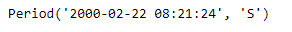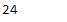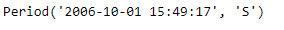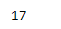Skip to content
Related Articles
Python | Pandas Period.second
• Last Updated : 06 Jan, 2019

Python is a great language for doing data analysis, primarily because of the fantastic ecosystem of data-centric python packages. Pandas is one of those packages and makes importing and analyzing data much easier.

Pandas` Period.second` attribute returns an integer value which represents the value of seconds in the given Period obeject.

Syntax : Period.second

Parameters : None

Return : seconds

Example #1: Use `Period.second `attribute to find the value of seconds present in the given Period object.

 `# importing pandas as pd``import` `pandas as pd`` ` `# Create the Period object``prd ``=` `pd.Period(freq ``=``'S'``, year ``=` `2000``, month ``=` `2``, day ``=` `22``,``                         ``hour ``=` `8``, minute ``=` `21``, second ``=` `24``)`` ` `# Print the Period object``print``(prd)`

Output :Now we will use the `Period.second` attribute to find the number of second in the given period object.

 `# return the number of seconds``prd.second`

Output :As we can see in the output, the `Period.second `attribute has returned 24 indicating that the number of seconds in prd object is 24.

Example #2: Use `Period.second` attribute to find the value of seconds present in the given Period object.

 `# importing pandas as pd``import` `pandas as pd`` ` `# Create the Period object``prd ``=` `pd.Period(freq ``=``'S'``, year ``=` `2006``, month ``=` `10``, ``               ``hour ``=` `15``, minute ``=` `49``, second ``=` `17``)`` ` `# Print the Period object``print``(prd)`

Output :Now we will use the `Period.second` attribute to find the number of second in the given period object.

 `# return the number of seconds``prd.second`

Output :As we can see in the output, the `Period.second `attribute has returned 17 indicating that the number of seconds in prd object is 17.

Attention geek! Strengthen your foundations with the Python Programming Foundation Course and learn the basics.

To begin with, your interview preparations Enhance your Data Structures concepts with the Python DS Course. And to begin with your Machine Learning Journey, join the Machine Learning – Basic Level Course

My Personal Notes arrow_drop_up